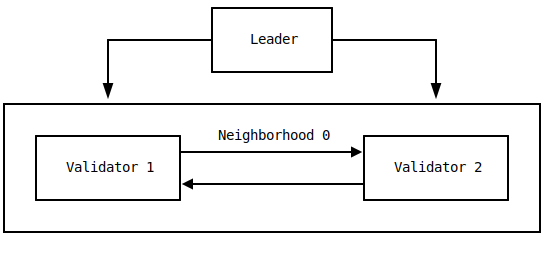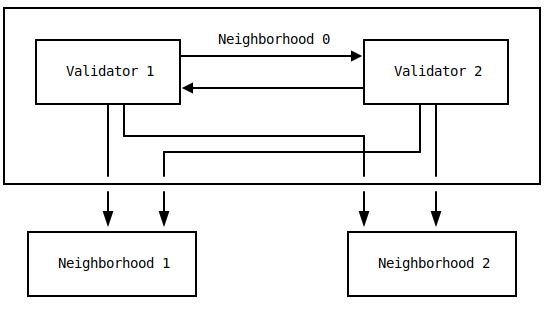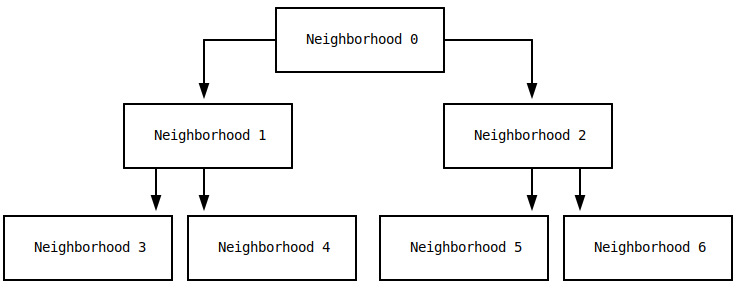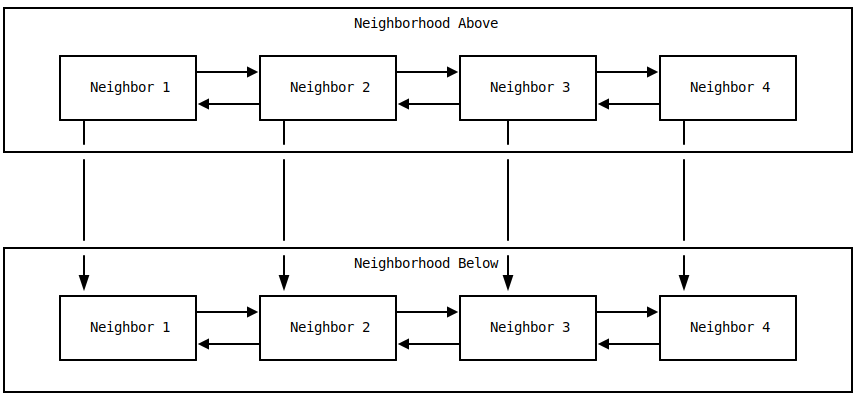Saltar al contenido principal

# Turbine Block Propagation

A Solana cluster uses a multi-layer block propagation mechanism called Turbine to broadcast transaction shreds to all nodes with minimal amount of duplicate messages. The cluster divides itself into small collections of nodes, called neighborhoods. Each node is responsible for sharing any data it receives with the other nodes in its neighborhood, as well as propagating the data on to a small set of nodes in other neighborhoods. This way each node only has to communicate with a small number of nodes.

During its slot, the leader node distributes shreds between the validator nodes in the first neighborhood (layer 0). Each validator shares its data within its neighborhood, but also retransmits the shreds to one node in some neighborhoods in the next layer (layer 1). The layer-1 nodes each share their data with their neighborhood peers, and retransmit to nodes in the next layer, etc, until all nodes in the cluster have received all the shreds.

## Neighborhood Assignment - Weighted Selection#

In order for data plane fanout to work, the entire cluster must agree on how the cluster is divided into neighborhoods. To achieve this, all the recognized validator nodes (the TVU peers) are sorted by stake and stored in a list. This list is then indexed in different ways to figure out neighborhood boundaries and retransmit peers. For example, the leader will simply select the first nodes to make up layer 0. These will automatically be the highest stake holders, allowing the heaviest votes to come back to the leader first. Layer 0 and lower-layer nodes use the same logic to find their neighbors and next layer peers.

To reduce the possibility of attack vectors, each shred is transmitted over a random tree of neighborhoods. Each node uses the same set of nodes representing the cluster. A random tree is generated from the set for each shred using a seed derived from the leader id, slot and shred index.

## Layer and Neighborhood Structure#

The current leader makes its initial broadcasts to at most DATA_PLANE_FANOUT nodes. If this layer 0 is smaller than the number of nodes in the cluster, then the data plane fanout mechanism adds layers below. Subsequent layers follow these constraints to determine layer-capacity: Each neighborhood contains DATA_PLANE_FANOUT nodes. Layer 0 starts with 1 neighborhood with fanout nodes. The number of nodes in each additional layer grows by a factor of fanout.

As mentioned above, each node in a layer only has to broadcast its shreds to its neighbors and to exactly 1 node in some next-layer neighborhoods, instead of to every TVU peer in the cluster. A good way to think about this is, layer 0 starts with 1 neighborhood with fanout nodes, layer 1 adds fanout neighborhoods, each with fanout nodes and layer 2 will have fanout * number of nodes in layer 1 and so on.

This way each node only has to communicate with a maximum of 2 * DATA_PLANE_FANOUT - 1 nodes.

The following diagram shows how the Leader sends shreds with a fanout of 2 to Neighborhood 0 in Layer 0 and how the nodes in Neighborhood 0 share their data with each other.The following diagram shows how Neighborhood 0 fans out to Neighborhoods 1 and 2.Finally, the following diagram shows a two layer cluster with a fanout of 2.### Configuration Values#

DATA_PLANE_FANOUT - Determines the size of layer 0. Subsequent layers grow by a factor of DATA_PLANE_FANOUT. The number of nodes in a neighborhood is equal to the fanout value. Neighborhoods will fill to capacity before new ones are added, i.e if a neighborhood isn't full, it must be the last one.

Currently, configuration is set when the cluster is launched. In the future, these parameters may be hosted on-chain, allowing modification on the fly as the cluster sizes change.

## Calculating the required FEC rate#

Turbine relies on retransmission of packets between validators. Due to retransmission, any network wide packet loss is compounded, and the probability of the packet failing to reach its destination increases on each hop. The FEC rate needs to take into account the network wide packet loss, and the propagation depth.

A shred group is the set of data and coding packets that can be used to reconstruct each other. Each shred group has a chance of failure, based on the likelyhood of the number of packets failing that exceeds the FEC rate. If a validator fails to reconstruct the shred group, then the block cannot be reconstructed, and the validator has to rely on repair to fixup the blocks.

The probability of the shred group failing can be computed using the binomial distribution. If the FEC rate is 16:4, then the group size is 20, and at least 4 of the shreds must fail for the group to fail. Which is equal to the sum of the probability of 4 or more trails failing out of 20.

Probability of a block succeeding in turbine:

• Probability of packet failure: P = 1 - (1 - network_packet_loss_rate)^2
• FEC rate: K:M
• Number of trials: N = K + M
• Shred group failure rate: S = SUM of i=0 -> M for binomial(prob_failure = P, trials = N, failures = i)
• Shreds per block: G
• Block success rate: B = (1 - S) ^ (G / N)
• Binomial distribution for exactly i results with probability of P in N trials is defined as (N choose i) * P^i * (1 - P)^(N-i)

For example:

• Network packet loss rate is 15%.
• 50k tps network generates 6400 shreds per second.
• FEC rate increases the total shreds per block by the FEC ratio.

With a FEC rate: 16:4

• G = 8000
• P = 1 - 0.85 * 0.85 = 1 - 0.7225 = 0.2775
• S = SUM of i=0 -> 4 for binomial(prob_failure = 0.2775, trials = 20, failures = i) = 0.689414
• B = (1 - 0.689) ^ (8000 / 20) = 10^-203

With FEC rate of 16:16

• G = 12800
• S = SUM of i=0 -> 32 for binomial(prob_failure = 0.2775, trials = 64, failures = i) = 0.002132
• B = (1 - 0.002132) ^ (12800 / 32) = 0.42583

With FEC rate of 32:32

• G = 12800
• S = SUM of i=0 -> 32 for binomial(prob_failure = 0.2775, trials = 64, failures = i) = 0.000048
• B = (1 - 0.000048) ^ (12800 / 64) = 0.99045

## Neighborhoods#

The following diagram shows how two neighborhoods in different layers interact. To cripple a neighborhood, enough nodes (erasure codes +1) from the neighborhood above need to fail. Since each neighborhood receives shreds from multiple nodes in a neighborhood in the upper layer, we'd need a big network failure in the upper layers to end up with incomplete data.